# How to easily find the optimal solution without exhaustive search using Genetic Algorithms

Oct. 24, 2015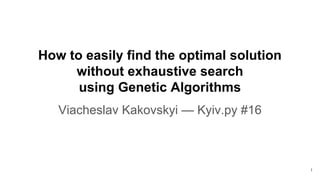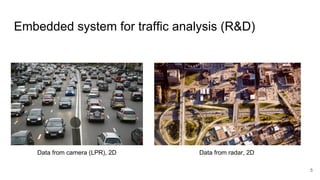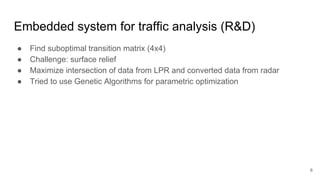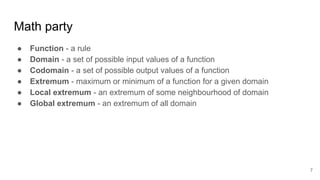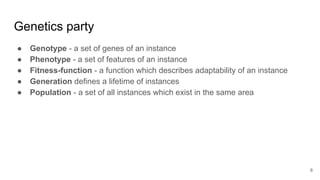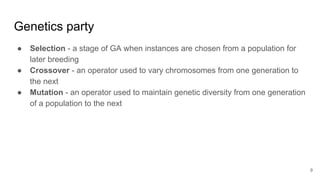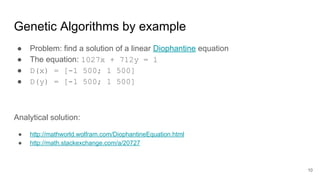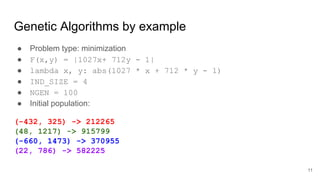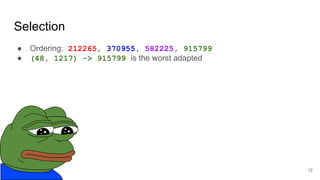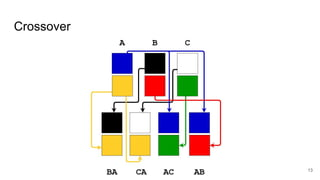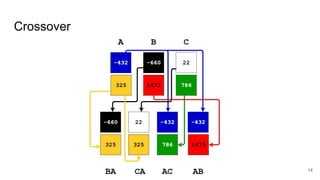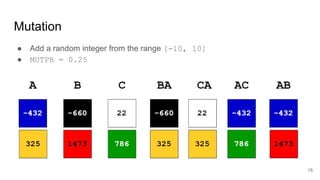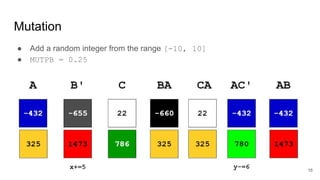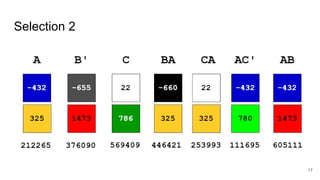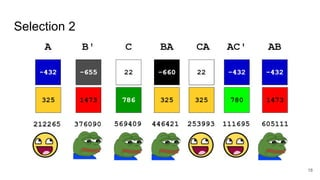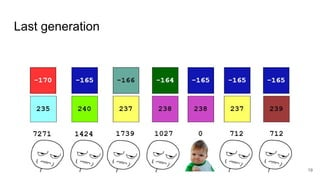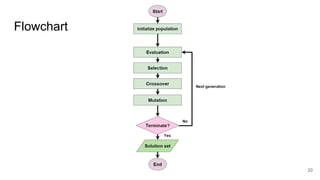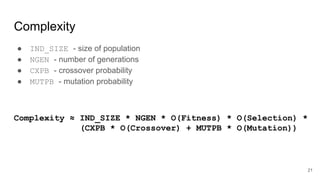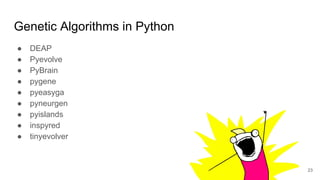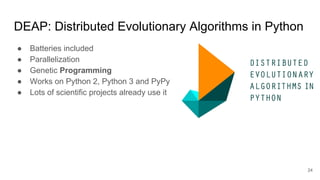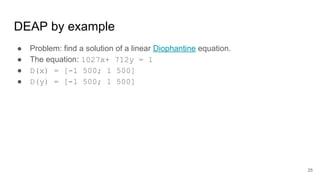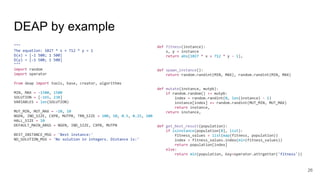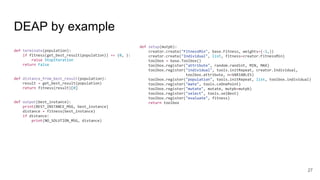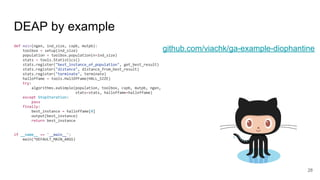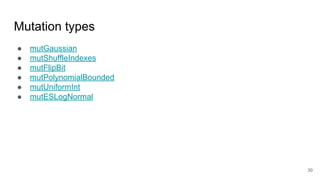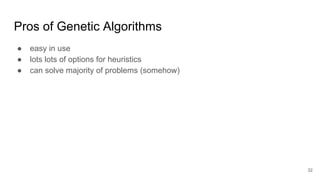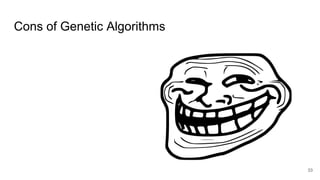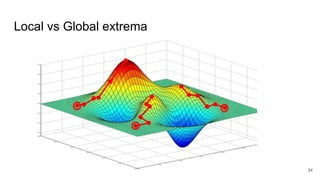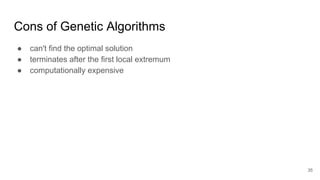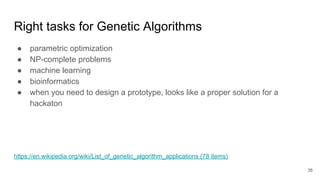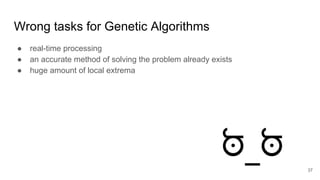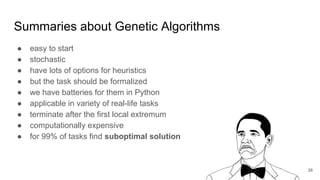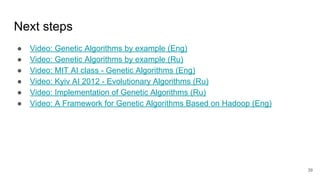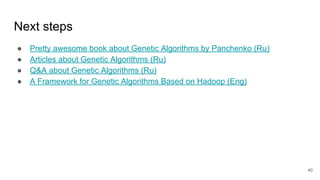1 of 43

### How to easily find the optimal solution without exhaustive search using Genetic Algorithms

• 1. How to easily find the optimal solution without exhaustive search using Genetic Algorithms Viacheslav Kakovskyi — Kyiv.py #16 1
• 2. Me! @kakovskyi Python Developer at SoftServe Recent projects: ● Backend for instant messenger ● Backend for embedded system for traffic analysis ● Software for personal nutrition planning 2
• 3. Agenda ● Cases from my experience ● Terminology ● Genetic Algorithms by example ● Theoretical background ● Genetic Algorithms in Python ● Pros and cons ● Tasks from real life ● Summary ● Further reading 3
• 4. Software for personal nutrition planning ● Find a set of suboptimal personal nutrition plans ● Minimize difference between required macronutrients and energetic value for suggested plan ● Genetic Algorithms used for generation of daily meals ● The problem is similar to Knapsack problem 4
• 5. Embedded system for traffic analysis (R&D) 5 Data from camera (LPR), 2D Data from radar, 2D
• 6. Embedded system for traffic analysis (R&D) ● Find suboptimal transition matrix (4x4) ● Challenge: surface relief ● Maximize intersection of data from LPR and converted data from radar ● Tried to use Genetic Algorithms for parametric optimization 6
• 7. Math party ● Function - a rule ● Domain - a set of possible input values of a function ● Codomain - a set of possible output values of a function ● Extremum - maximum or minimum of a function for a given domain ● Local extremum - an extremum of some neighbourhood of domain ● Global extremum - an extremum of all domain 7
• 8. Genetics party ● Genotype - a set of genes of an instance ● Phenotype - a set of features of an instance ● Fitness-function - a function which describes adaptability of an instance ● Generation defines a lifetime of instances ● Population - a set of all instances which exist in the same area 8
• 9. Genetics party ● Selection - a stage of GA when instances are chosen from a population for later breeding ● Crossover - an operator used to vary chromosomes from one generation to the next ● Mutation - an operator used to maintain genetic diversity from one generation of a population to the next 9
• 10. Genetic Algorithms by example ● Problem: find a solution of a linear Diophantine equation ● The equation: 1027x + 712y = 1 ● D(x) = [-1 500; 1 500] ● D(y) = [-1 500; 1 500] Analytical solution: ● http://mathworld.wolfram.com/DiophantineEquation.html ● http://math.stackexchange.com/a/20727 10
• 11. Genetic Algorithms by example ● Problem type: minimization ● F(x,y) = |1027x+ 712y - 1| ● lambda x, y: abs(1027 * x + 712 * y - 1) ● IND_SIZE = 4 ● NGEN = 100 ● Initial population: (-432, 325) -> 212265 (48, 1217) -> 915799 (-660, 1473) -> 370955 (22, 786) -> 582225 11
• 12. Selection ● Ordering: 212265, 370955, 582225, 915799 ● (48, 1217) -> 915799 is the worst adapted 12
• 15. Mutation 15 ● Add a random integer from the range [-10, 10] ● MUTPB = 0.25
• 16. Mutation 16 ● Add a random integer from the range [-10, 10] ● MUTPB = 0.25
• 21. Complexity ● IND_SIZE - size of population ● NGEN - number of generations ● CXPB - crossover probability ● MUTPB - mutation probability Complexity ≈ IND_SIZE * NGEN * O(Fitness) * O(Selection) * (CXPB * O(Crossover) + MUTPB * O(Mutation)) 21
• 22. Guidelines ● Define your fitness-function ● Choose the problem type ● Define the domain and NGEN ● Define how the initial population should be chosen and IND_SIZE ● Define the type of selection ● Define the type of crossover ● Define the type of mutation and MUTPB ● Try it! ● Define heuristics if needed 22
• 23. Genetic Algorithms in Python ● DEAP ● Pyevolve ● PyBrain ● pygene ● pyeasyga ● pyneurgen ● pyislands ● inspyred ● tinyevolver 23
• 24. DEAP: Distributed Evolutionary Algorithms in Python ● Batteries included ● Parallelization ● Genetic Programming ● Works on Python 2, Python 3 and PyPy ● Lots of scientific projects already use it 24
• 25. DEAP by example ● Problem: find a solution of a linear Diophantine equation. ● The equation: 1027x+ 712y = 1 ● D(x) = [-1 500; 1 500] ● D(y) = [-1 500; 1 500] 25
• 26. DEAP by example """ The equation: 1027 * x + 712 * y = 1 D(x) = [-1 500; 1 500] D(y) = [-1 500; 1 500] """ import random import operator from deap import tools, base, creator, algorithms MIN, MAX = -1500, 1500 SOLUTION = [-165, 238] VARIABLES = len(SOLUTION) MUT_MIN, MUT_MAX = -10, 10 NGEN, IND_SIZE, CXPB, MUTPB, TRN_SIZE = 100, 50, 0.5, 0.25, 100 HALL_SIZE = 10 DEFAULT_MAIN_ARGS = NGEN, IND_SIZE, CXPB, MUTPB BEST_INSTANCE_MSG = 'Best instance:' NO_SOLUTION_MSG = 'No solution in integers. Distance is:' 26 def fitness(instance): x, y = instance return abs(1027 * x + 712 * y - 1), def spawn_instance(): return random.randint(MIN, MAX), random.randint(MIN, MAX) def mutate(instance, mutpb): if random.random() <= mutpb: index = random.randint(0, len(instance) - 1) instance[index] += random.randint(MUT_MIN, MUT_MAX) return instance, return instance, def get_best_result(population): if isinstance(population, list): fitness_values = list(map(fitness, population)) index = fitness_values.index(min(fitness_values)) return population[index] else: return min(population, key=operator.attrgetter('fitness'))
• 27. DEAP by example def terminate(population): if fitness(get_best_result(population)) == (0, ): raise StopIteration return False def distance_from_best_result(population): result = get_best_result(population) return fitness(result) def output(best_instance): print(BEST_INSTANCE_MSG, best_instance) distance = fitness(best_instance) if distance: print(NO_SOLUTION_MSG, distance) 27 def setup(mutpb): creator.create("FitnessMin", base.Fitness, weights=(-1,)) creator.create("Individual", list, fitness=creator.FitnessMin) toolbox = base.Toolbox() toolbox.register("attribute", random.randint, MIN, MAX) toolbox.register("individual", tools.initRepeat, creator.Individual, toolbox.attribute, n=VARIABLES) toolbox.register("population", tools.initRepeat, list, toolbox.individual) toolbox.register("mate", tools.cxOnePoint) toolbox.register("mutate", mutate, mutpb=mutpb) toolbox.register("select", tools.selBest) toolbox.register("evaluate", fitness) return toolbox
• 28. DEAP by example def main(ngen, ind_size, cxpb, mutpb): toolbox = setup(ind_size) population = toolbox.population(n=ind_size) stats = tools.Statistics() stats.register("best_instance_of_population", get_best_result) stats.register("distance", distance_from_best_result) stats.register("terminate", terminate) halloffame = tools.HallOfFame(HALL_SIZE) try: algorithms.eaSimple(population, toolbox, cxpb, mutpb, ngen, stats=stats, halloffame=halloffame) except StopIteration: pass finally: best_instance = halloffame output(best_instance) return best_instance if __name__ == '__main__': main(*DEFAULT_MAIN_ARGS) 28 github.com/viachk/ga-example-diophantine
• 29. Crossover types 29 ● cxOnePoint ● cxTwoPoint ● cxUniform ● cxPartialyMatched ● cxUniformPartialyMatched ● cxOrdered ● cxBlend ● cxESBlend ● cxESTwoPoint ● cxSimulatedBinary ● cxSimulatedBinaryBounded ● cxMessyOnePoint
• 30. Mutation types ● mutGaussian ● mutShuffleIndexes ● mutFlipBit ● mutPolynomialBounded ● mutUniformInt ● mutESLogNormal 30
• 31. Selection types ● selTournament ● selRoulette ● selNSGA2 ● selSPEA2 ● selRandom ● selBest ● selWorst ● selTournamentDCD ● selDoubleTournament 31
• 32. Pros of Genetic Algorithms ● easy in use ● lots lots of options for heuristics ● can solve majority of problems (somehow) 32
• 33. Cons of Genetic Algorithms 33
• 34. Local vs Global extrema 34
• 35. Cons of Genetic Algorithms ● can't find the optimal solution ● terminates after the first local extremum ● computationally expensive 35
• 36. Right tasks for Genetic Algorithms ● parametric optimization ● NP-complete problems ● machine learning ● bioinformatics ● when you need to design a prototype, looks like a proper solution for a hackaton https://en.wikipedia.org/wiki/List_of_genetic_algorithm_applications (78 items) 36
• 37. Wrong tasks for Genetic Algorithms ● real-time processing ● an accurate method of solving the problem already exists ● huge amount of local extrema 37
• 38. Summaries about Genetic Algorithms ● easy to start ● stochastic ● have lots of options for heuristics ● but the task should be formalized ● we have batteries for them in Python ● applicable in variety of real-life tasks ● terminate after the first local extremum ● computationally expensive ● for 99% of tasks find suboptimal solution 38
• 39. Next steps ● Video: Genetic Algorithms by example (Eng) ● Video: Genetic Algorithms by example (Ru) ● Video: MIT AI class - Genetic Algorithms (Eng) ● Video: Kyiv AI 2012 - Evolutionary Algorithms (Ru) ● Video: Implementation of Genetic Algorithms (Ru) ● Video: A Framework for Genetic Algorithms Based on Hadoop (Eng) 39
• 40. Next steps ● Pretty awesome book about Genetic Algorithms by Panchenko (Ru) ● Articles about Genetic Algorithms (Ru) ● Q&A about Genetic Algorithms (Ru) ● A Framework for Genetic Algorithms Based on Hadoop (Eng) 40